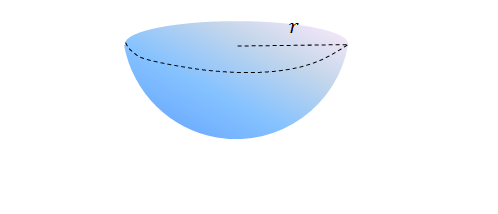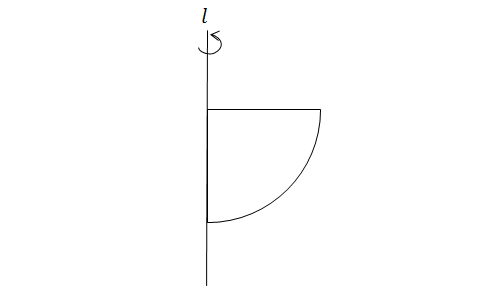Geometry

# Surface Area - Sphere

The surface area of a sphere with radius $5$ is equal to $M \pi$, where $M$ is a positive integer. Determine the value of $M$.

Given a sphere with volume $\frac{32}{3}\pi$, what is the surface area of the sphere?Given a solid hemisphere with radius $12,$ what is the total surface area of the hemisphere?

Given a sphere with radius $7$ and surface area $A$, if the radius of the sphere is increased to $2 \times 7$, and the resulting surface area is $c A$, what is the value of $c$?The above diagram shows a quarter circle with radius $10.$ If the quarter circle is rotated $360^\circ$ around line $l,$ what is the surface area of the resulting body?

×